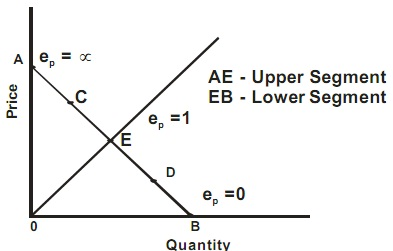#### Point method

Point method:

We can evaluate the price elasticity of demand at a point on linear demand curve. The formula to find out ep through point method is,For illustration, in figure above, the length of the demand curve AB is 4 cm.

Accurately at the middle point of AB demand curve,

A)  ep at point e:

ep = EB/EA = 2/2  = 1  ∴  ep = 1

B) ep at point D = (middle point of EB part of demand curve)

ep = DB/DA = 1/3 = 0.3 ∴ ep < 1

C) ep at point c (middle point of EA part of demand curve)

ep = CB/CA =  3/1 =  3  ∴ ep > 1

D) ep at point B = 0/AB = 0

(i.e., o by anything is zero, a mathematical principle)    ∴ ep = 0

E) ep at point A = AB/0 = ∞

(That is, anything divided by zero becomes infinity a mathematical principle)
∴ ep =  ∞

Latest technology based Economics Online Tutoring Assistance

Tutors, at the www.tutorsglobe.com, take pledge to provide full satisfaction and assurance in Theory of Demand help via online tutoring. Students are getting 100% satisfaction by online tutors across the globe. Here you can get homework help for Theory of Demand, project ideas and tutorials. We provide email based Theory of Demand help. You can join us to ask queries 24x7 with live, experienced and qualified online tutors specialized in Theory of Demand. Through Online Tutoring, you would be able to complete your homework or assignments at your home. Tutors at the TutorsGlobe are committed to provide the best quality online tutoring assistance for Economics Homework help and assignment help services. They use their experience, as they have solved thousands of Economics assignments, which may help you to solve your complex issues of Theory of Demand. TutorsGlobe assure for the best quality compliance to your homework. Compromise with quality is not in our dictionary. If we feel that we are not able to provide the homework help as per the deadline or given instruction by the student, we refund the money of the student without any delay.